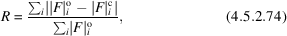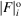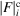International
Tables for
Crystallography
Volume B
Reciprocal space
Edited by U. Shmueli

International Tables for Crystallography (2010). Vol. B, ch. 4.5, pp. 582-583   | 1 | 2 |

## Section 4.5.2.6.8. Reliability

R. P. Millanea

#### 4.5.2.6.8. Reliability

| top | pdf |

As with structure determination in any area of crystallography, assessment of the reliability or precision of a structure is critically important. The most commonly used measure of reliability in fibre diffraction is the R factor, calculated aswhereanddenote the observed (measured) and calculated, respectively, amplitude of either the samples (along R) of the cylindrically averaged intensity(for a noncrystalline specimen) or the cylindrically averaged structure factors(for a polycrystalline specimen). One way of assessing the significance of the R factor obtained in a particular structure determination is by comparing it with the `largest likely R factor' (Wilson, 1950), i.e. the expected value of the R factor for a random distribution of atoms. Wilson (1950)showed that the largest likely R factor is 0.83 for a centric crystal and 0.59 for an acentric crystal. Although it does not provide a quantitative measure of structural reliability, the largest likely R factor does provide a useful yardstick for evaluating the significance of R factors obtained in structure determinations.

The largest likely R factor for fibre diffraction can be calculated from the amplitude statistics, which depend on the number of degrees of freedom, m, in the measured intensity (Stubbs, 1989; Millane, 1990a). Making use of these statistics shows that the largest likely R factor,, for m components is given by (Stubbs, 1989; Millane, 1989a)whereis the binomial coefficient andthe incomplete beta function. The beta function in equation (4.5.2.75)can be replaced by a finite series that is easy to evaluate (Millane, 1989a). The expression in equation (4.5.2.75)forcan be written in various approximate forms (Millane, 1990d, 1992a), the simplest being(Millane, 1990d), which shows that the largest likely R factor falls off approximately aswith increasing m. This is because it is easier to match the sum of a number of structure amplitudes than to match each of them individually. The important conclusion is that the largest likely R factor is smaller in fibre diffraction than in conventional crystallography (whereor 2), and it is smaller when there are more overlapping reflections. This means that for equivalent precision, the R factor must be smaller for a structure determined by fibre diffraction than for one determined by conventional crystallography. How much smaller depends on the number of overlapping reflections on the diffraction pattern.

In a structure determination, the data have different values of m at different positions on the diffraction pattern. Using the definition of the R factor, equation (4.5.2.74), shows that the largest likely R factor for a structure determination is given by (Millane, 1989b)where the sums are over the values of m on the diffraction pattern,is the number of data that have m components,is given by equation (4.5.2.75)andis given bywhereis the gamma function. The quantities on the right-hand side of equation (4.5.2.77)are easily determined for a particular data set. The largest likely R factor decreases (since m increases) with increasing resolution of the data, increasing diameter of the molecule and decreasing order u of the helix symmetry. For example, for TMV at 5 Å resolution the largest likely R factor is 0.37, and at 3 Å resolution it is 0.31, whereas for a tenfold nucleic acid structure at 3 Å resolution it is 0.40 (Millane, 1989b, 1992b). This underlines the importance of comparing R factors obtained in a fibre diffraction analysis with the largest likely R factor; an R factor of 0.25 that may indicate a good protein structure may, or may not, indicate a well determined fibre structure.

Using approximations for,and m allows the following approximation for the largest likely R factor for a noncrystalline fibre to be derived (Millane, 1992b):whereis the resolution of the data. The approximation (4.5.2.79)is generally not good enough for calculating accurate largest likely R factors, but it does show the general behaviour with helix symmetry, molecular diameter and diffraction-data resolution. Other approximations to largest likely R factors have been derived that are quite accurate and also include the effect of a minimum resolution for the data (Millane, 1992b).

Largest likely R factors in fibre diffraction studies are typically between about 0.3 and 0.5, depending on the particular structure (Millane, 1989b, 1992b; Millane & Stubbs, 1992). Although the largest likely R factor does not give a quantitative assessment of the significance of an R factor obtained in a particular structure determination, it can be used as a guide to the significance. R factors obtained for well determined protein structures are typically between about one-third and one-half of the corresponding largest likely R factor, depending on the resolution. It is therefore reasonable to expect the R factor for a well determined fibre structure to be between one-third and one-half of the largest likely R factor calculated for the structure. R factors should, therefore, generally be less than 0.15 to 0.25, depending on the particular structure and the resolution as illustrated by the examples presented in Millane & Stubbs (1992).

The free R factor (Brünger, 1997) has become popular in single-crystal crystallography as a tool for validation of refinements. The free R factor is more difficult to implement (but is probably even more important) in fibre diffraction studies because of the smaller data sets, but has been used to advantage in recent studies (Hudson et al., 1997; Welsh et al., 1998, 2000).

### References

Brünger, A. T. (1997). Free R value: cross-validation in crystallography. Methods Enzymol. 277, 366–396.Google Scholar
Hudson, L., Harford, J. J., Denny, R. C. & Squire, J. M. (1997). Myosin head configuration in relaxed fish muscle: resting state myosin heads must swing axially by up to 150 Å or turn upside down to reach rigor. J. Mol. Biol. 273, 440–455.Google Scholar
Millane, R. P. (1989a). R factors in X-ray fibre diffraction. I. Largest likely R factors for N overlapping terms. Acta Cryst. A45, 258–260.Google Scholar
Millane, R. P. (1989b). R factors in X-ray fibre diffraction. II. Largest likely R factors. Acta Cryst. A45, 573–576.Google Scholar
Millane, R. P. (1990a). Intensity distributions in fibre diffraction. Acta Cryst. A46, 552–559.Google Scholar
Millane, R. P. (1990d). R factors in X-ray fibre diffraction. III. Asymptotic approximations to largest likely R factors. Acta Cryst. A46, 68–72.Google Scholar
Millane, R. P. (1992a). Largest likely R factors for normal distributions. Acta Cryst. A48, 649–650.Google Scholar
Millane, R. P. (1992b). R factors in X-ray fibre diffraction. IV. Analytic expressions for largest likely R factors. Acta Cryst. A48, 209–215.Google Scholar
Millane, R. P. & Stubbs, G. (1992). The significance of R factors in fibre diffraction. Polym. Prepr. 33, 321–322.Google Scholar
Stubbs, G. (1989). The probability distributions of X-ray intensities in fibre diffraction: largest likely values for fibre diffraction R factors. Acta Cryst. A45, 254–258.Google Scholar
Welsh, L. C., Symmons, M. F. & Marvin, D. A. (2000). The molecular structure and structural transition of the α-helical capsid in filamentous bacteriophage Pf1. Acta Cryst. D56, 137–150.Google Scholar
Welsh, L. C., Symmons, M. F., Sturtevant, J. M., Marvin, D. A. & Perham, R. N. (1998). Structure of the capsid of Pf3 filamentous phage determined from X-ray fiber diffraction data at 3.1 Å resolution. J. Mol. Biol. 283, 155–177.Google Scholar
Wilson, A. J. C. (1950). Largest likely values for the reliability index. Acta Cryst. 3, 397–399.Google Scholar